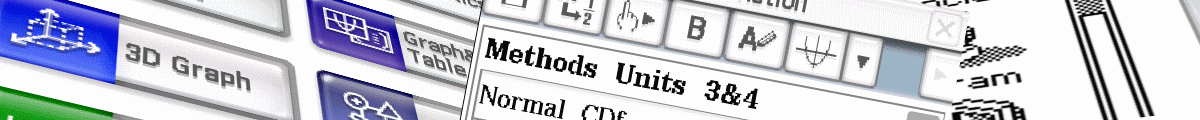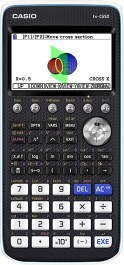## Casio fx-CG 10/20/50 AU - Home

Heaps of free resources for Casio fx CG 10, 20, 50 and AU models on these new pages and more coming! Make sure you read our download guide for help with installing programs and eActivities into your calculator. If you're using the fx-CG Manager, read this guide instead.The Casio fxCG50 is a colour graphics calculator that operates in the same way as earlier fxCG20 and fx9860G models but has a faster processor.

Inbuilt USB mass storage means that data transfer between the calculator and a computer is simple - plug in the calculator and hit F1 for it to behave like a USB drive.

Standard apps that the calculator ships with:

• Run-Matrix: General computation.
• Statistics: single-variable and paired variable stats, tests, intervals, etc.
• eActivities: Notebook-like interface to store text and formulas or create copies of apps.
• Graph: Draw, store and calculate with functions.
• Dynamic Graph: Draw multiple versions of a functions.
• Table: Generate table of values for a function and then plot.
• Recursion: Generate table of values and draw graphs.
• Conic Graph: Graph and explore conic sections.
• Equation: Tool for solving systems of equations and polynomials.
• Program: Run imported programs or write your own code to enhance calculator capability.
• Financial: Calculate loan repayments, compound interest, annuities, etc.
• E-Con4: Manage a data logger such as Casio EA-200.
• Link: Use to swap data with another calculator.
• Memory: Manage data memory.

• Probability Simulation: Simulate coin toss, dice roll, spinner, marble grab, card draw and random numbers.
• Physium: Display periodic table of elements and fundamental physical constants.
• Picture Plot: Plot points on pictures and analysis.
• Geometry: Draw and Analyze geometric objects.
• Conversion: Convert values from one unit of measurement to another.
• 3D Graph: Graph a line, plane, sphere, cylinder or cone in 3D

QCE Sample Methods Exam Using fxCG50

Here are 5 YouTube videos showing ways to use the fxCG50 in the QCE sample external Methods U3&4 exam. Thanks to Alistair!

QAMTAC18

### News

Free and paid tutorials at ClassPad Help and the Tuition Centre sites.